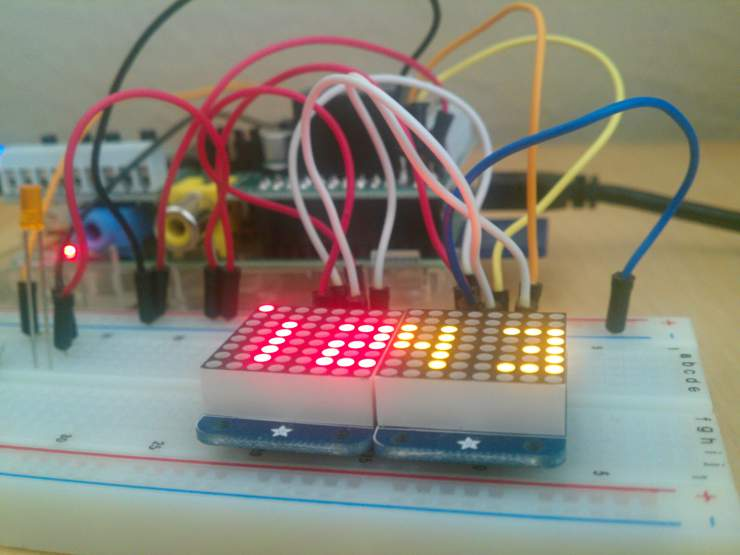As my first RaspberryPi Project I decided to build a clock using the two LED dot matrices I bought. In addition to providing a quick glance at the time I wanted to be able to quickly tell if I had any new unread emails. Thus the emClock was conceived!

My first goal was to build the clock!

The two LED dot matrices I bought came with an I2C compatible interface which made set up and programming infinitely times easier than having to solder and multiplex 64 LEDs by myself. Enabling I2C on the RPI is a breeze as there are many great tutorials out there for how to do it. To access them programmatically them I used Adafruit’s LED Backpack python library.

After setting up the LED matrices I had to create a framework for displaying numbers. I created a an array of matrices which represent the LED’s that are powered for each number. For example number zero is defined as:

``````n0 = [[1,1,1],
[1,0,1],
[1,0,1],
[1,0,1],
[1,1,1]]
``````

This allowed me to write a generic plotNumber function which takes two parameters: which number to display, and where to display it

``````def plotNum(c,n):
if c<2:
wgrid = grid
else:
wgrid = grid2

for ix, x in enumerate(nums[n]):
for iy, y in enumerate(x):
if y==1:
wgrid.setPixel(iy+((c%2)*5),ix+2)
``````

Once I created a framework for displaying the numbers, building the actual time-keeping function was easy.

``````pastMinute = 0
def clockStep():
global pastMinute
now = datetime.datetime.now()
hour = now.hour
minute = now.minute
second = now.second

if minute != pastMinute:
pastMinute = minute
grid.clear()
grid2.clear()

plotNum(0,hour/10)
plotNum(1,hour%10)

plotNum(2,minute/10)
plotNum(3,minute%10)
else:
if (second<32):
grid.setPixel(second/4,0)
else:
grid2.setPixel((second-32)/4,0)
``````

In order to keep time, I create a thread which runs `clockStep()` every second using a threading helper function from StackOverflow

``````def do_every(interval, worker_func, iterations = 0):
if iterations != 1:
interval,
do_every, [interval, worker_func, 0 if iterations == 0 else iterations-1]
).start();
worker_func();
``````

Once the clock portion was done, it was time to add the email checking functionality! Now, I am a Google fanboy so naturally I used Gmail, which conveniently provides an RSS feed for your emails which can be accessed at https://USERNAME:PASSWORD@mail.google.com/gmail/feed/atom.

Armed with this knowledge, we can proceed to write our emailCheck() function!

``````pastEmails = 0
def emailCheck():
global pastEmails
if emails > pastEmails:
pastEmails = emails
notifySound(emails)
led(7,1)
elif emails < 2:
led(7,0)
``````

We can invoke emailCheck() and clockStep() using our threading helper function

``````do_every(1,clockStep)
do_every(10,emailCheck)
``````

And thus emClock was born! :D